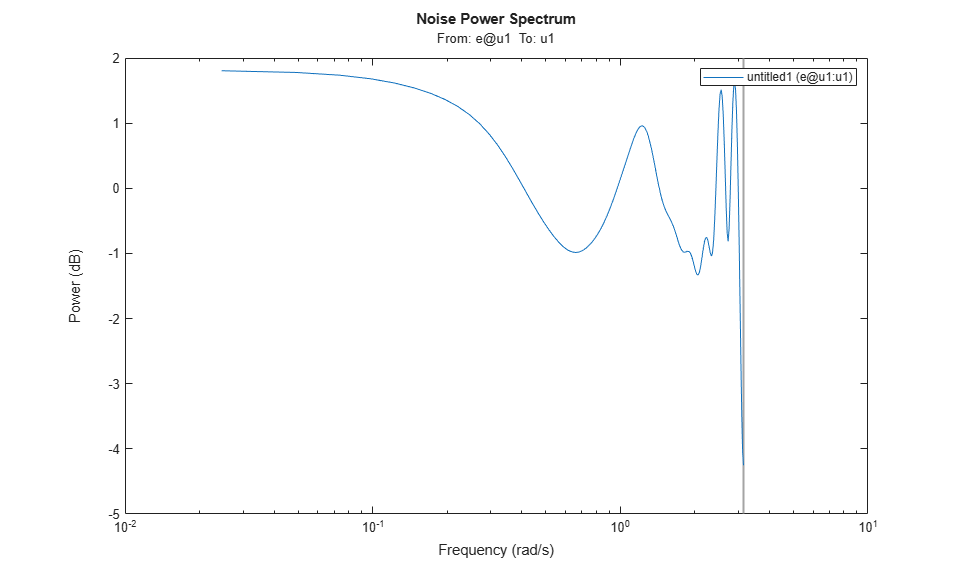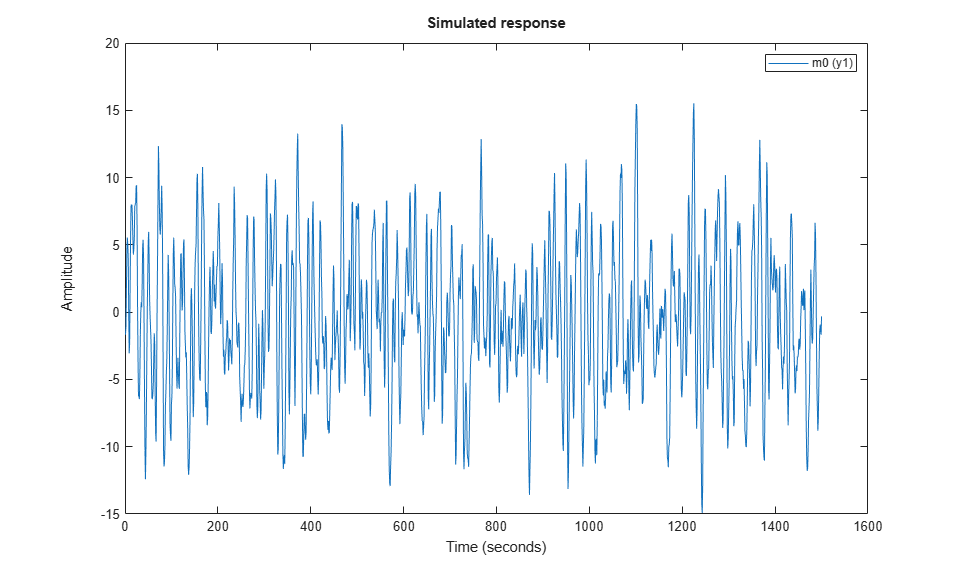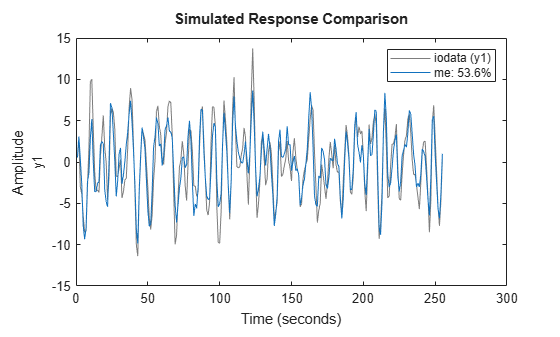## Generate Data Using Simulation

### Commands for Generating Data Using Simulation

You can generate input data and then use it with a model to create output data.

Simulating output data requires that you have a model with known coefficients. For more information about commands for constructing models, see Commands for Constructing Linear Model Structures.

To generate input data, use `idinput` to construct a signal with the desired characteristics, such as a random Gaussian or binary signal or a sinusoid. `idinput` returns a matrix of input values.

The following table lists the commands you can use to simulate output data. For more information about these commands, see the corresponding reference pages.

Commands for Generating Data

CommandDescriptionExample
`idinput`Constructs a signal with the desired characteristics, such as a random Gaussian or binary signal or a sinusoid, and returns a matrix of input values.
```u = iddata([],... idinput(400,'rbs',[0 0.3])); ```
`sim`Simulates response data based on existing linear or nonlinear parametric model in the MATLAB® workspace.

To simulate the model output `y` for a given input, use the following command:

`y = sim(m,data)`

`m` is the model object name, and `data` is input data matrix or `iddata` object.

### Create Periodic Input Data

This example shows how to create a periodic random Gaussian input signal using `idinput`.

Create a periodic input for one input and consisting of five periods, where each period is 300 samples.

```per_u = idinput([300 1 5]); ```

Create an `iddata` object using the periodic input and leaving the output empty.

```u = iddata([],per_u,'Period',.300); ```

View the data characteristics in time- and frequency-domain.

```% Plot data in time-domain. plot(u) % Plot the spectrum. spectrum(spa(u)) ```(Optional) Simulate model output using the data.

```% Construct a polynomial model. m0 = idpoly([1 -1.5 0.7],[0 1 0.5]); % Simulate model output with Gaussian noise. sim_opt = simOptions('AddNoise',true); sim(m0,u,sim_opt) ```### Generate Output Data Using Simulation

This example shows how to generate output data by simulating a model using an input signal created using `idinput`.

You use the generated data to estimate a model of the same order as the model used to generate the data. Then, you check how closely both models match to understand the effects of input data characteristics and noise on the estimation.

Create an ARMAX model with known coefficients.

```A = [1 -1.2 0.7]; B = {[0 1 0.5 0.1],[0 1.5 -0.5],[0 -0.1 0.5 -0.1]}; C = [1 0 0 0 0]; Ts = 1; m0 = idpoly(A,B,C,'Ts',1);```

The leading zeros in the `B` matrix indicate the input delay (`nk`), which is `1` for each input channel.

Construct a pseudorandom binary input data.

`u = idinput([255,3],'prbs');`

Simulate model output with noise using the input data.

`y = sim(m0,u,simOptions('AddNoise',true));`

Represent the simulation data as an `iddata` object.

`iodata = iddata(y,u,m0.Ts);`

(Optional) Estimate a model of the same order as `m0` using `iodata`.

```na = 2; nb = [3 2 3]; nc = 4; nk = [1 1 1]; me = armax(iodata,[na,nb,nc,nk]);```

Use `bode(m0,me)` and `compare(iodata,me)` to check how closely `me` and `m0` match.

`compare(iodata,me);`### Simulating Data Using Other MathWorks Products

You can also simulate data using the Simulink® and Signal Processing Toolbox™ software. Data simulated outside the System Identification Toolbox™ product must be in the MATLAB workspace as double matrices. For more information about simulating models using the Simulink software, see Simulate Identified Model in Simulink.

## SupportGet trial now